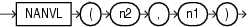NANVL

SyntaxDescription of the illustration nanvl.eps

Purpose

The NANVL function is useful only for floating-point numbers of type BINARY_FLOAT or BINARY_DOUBLE. It instructs Oracle Database to return an alternative value n1 if the input value n2 is NaN (not a number). If n2 is not NaN, then Oracle returns n2.

This function takes as arguments any numeric data type or any nonnumeric data type that can be implicitly converted to a numeric data type. Oracle determines the argument with the highest numeric precedence, implicitly converts the remaining arguments to that data type, and returns that data type.

Table 2-8 for more information on implicit conversion, for information on binary-float comparison semantics, and for information on numeric precedence

Examples

Using table float_point_demo created for TO_BINARY_DOUBLE, insert a second entry into the table:

INSERT INTO float_point_demo
VALUES (0,'NaN','NaN');

SELECT *
FROM float_point_demo;

DEC_NUM BIN_DOUBLE  BIN_FLOAT
---------- ---------- ----------
1234.56 1.235E+003 1.235E+003
0        Nan        Nan

The following example returns bin_float if it is a number. Otherwise, 0 is returned.

SELECT bin_float, NANVL(bin_float,0)
FROM float_point_demo;

BIN_FLOAT NANVL(BIN_FLOAT,0)
---------- ------------------
1.235E+003         1.235E+003
Nan                  0